# Texas Go Math Grade 6 Lesson 4.1 Answer Key Multiplying Decimals

Refer to our Texas Go Math Grade 6 Answer Key Pdf to score good marks in the exams. Test yourself by practicing the problems from Texas Go Math Grade 6 Lesson 4.1 Answer Key Multiplying Decimals.

## Texas Go Math Grade 6 Lesson 4.1 Answer Key Multiplying Decimals

Use decimal grids or area models to find each product.

(A) 0.3 × 0.5
0.3 × 0.5 represents 0.3 of 0.5.
Use a decimal grid. Shade 5 rows of the grid to represent 0.5.
This represents ________ hundredth(s), or 0.15.
0.3 × 0.5 = _____________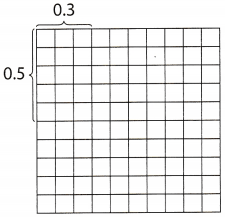(B) 3.2 × 2.1 ______
Use an area model. Each row contains 3 wholes + 2 tenths.
Each column contains _________ whole(s) + ________ tenth(s).
The entire area model represents
________ whole(s) + ________ tenth(s) + ________ hundredth(s).
3.2 × 2.1 = ________Reflect

Question 1.
Analyze Relationships How are the products 2.1 × 3.2 and 21 × 32 alike? How are they different?
The products of 2.1 × 3.2 and 21 × 32 will be the number with the same digits, but at 2.1 × 3.2 the result will has two decimal places and at 21 × 32 the result will be whole number, that is the difference.
Products

Products with the same digits, but product of 2.1 × 3.2 will has two decimal places while product of 21 × 32 will be whole number

Question 2.
Communicate Mathematical Ideas How can you use estimation to check that you have placed the decimal point correctly in your product?
We can check it multiplying whole numbers which are the nearest to the given decimals. The result suppose to be close to the right result.
We can check it multiplying whole numbers which are the nearest to the given decimals.

Multiply.

Question 3.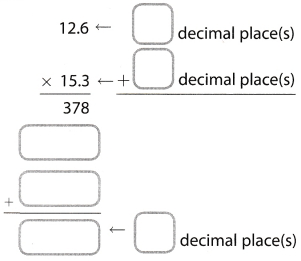Here, in the first factor we have one decimal place as well as in the second factor. So, product will have two decimal places. We have the following: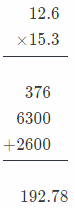So, the result is 192.78

Question 4.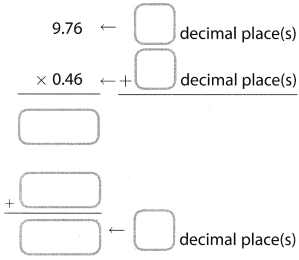In both factors there are two decimal places, so, the product will have four decimal places We have the following: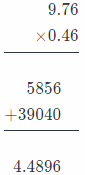So, the product is 4.4896

Multiply

Question 5.In both factors there are two decimal places, so, the product will have four decimal places We have the following:So, the product is 48.4092

Question 6.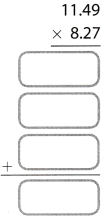In both factors there are two decimal places, so, the product will have four decimal places We have the following: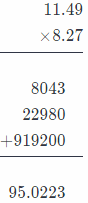So, the product is 95.0223

Question 7.
Rico bicycles at an average speed of 15.5 miles per hour.
What distance will Rico bicycle in 2.5 hours? ___________ miles
In order to find what distance Roco will bicycle in 2.5 hours multiplying 15.5 by 2.5. First factor has one decimal place, the second has one decimal place, so, the product will has two decimal places.

both factors there are two decimal places, so, the product will have four decimal places. We have the following: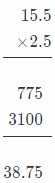Conclusion is that Roco will bicycle 38.75 miles in 2.5 hours

Question 8.
We can multiply 15 by 2 and get 30. After that, we can multiply 16 by 3 and get 48 We can add 30 and 48 and we get 78. After this, we can divide 78 by 2 and get 39.

So, the answer is reasonable because 39 is close to 38.75.
Multiply 15 by 2.16 by 3 and sum the product. Divide that sum by 2.

Question 1.
Use the grid to multiply 0.4 × 0.7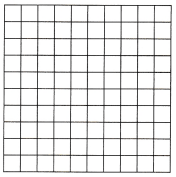0.4 × 0.7 = _______________
0.4 × 0.7 represents 0.4 of 0.7. We will use a decimal grid and shade 7 rows of the grid to represent 0.7. Now, we will shade 0.4 of each 0.1 that is already shaded to represent 0.4 of 1. So, now we have 28 squares which are double-shaded. This actually represents 28 hundredths, or 28. So, we have the following:
0.4 × 0.7 = 0.28Question 2.
Draw an area model to multiply 1.1 × 2.4

1.1 × 2.4 _______________
We will use an area model. Here, each row contains 1 whole + 1 tenth. Also, each column contains 2 wholes + 4 tenths. So, the entire area model represents:
2 wholes + 6 tenths + 4 hundreths.

Conclusion is that:
1.1 × 2.4 = 2.64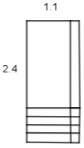Multiply.

Question 3.
0.18 × 0.06 = _______________
In both factors there are two decimal places, so, the product will have four decimal places We have the following:So, the product is 0.0108

Question 4.
35.15 × 3.7 = _______________
Here, in the first factor we have two decimal. places, but in the second we have one decimal. place, so, the product will have three decimal places.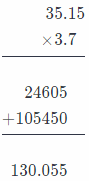So, the result is 130.055

Question 5.
0.96 × 0.12 = _______________
In both factors there are two decimal places, so, the product will have four decimal places. We have the following:So, the product is 0.1152

Question 6.
62.19 × 32.5 = _______________
In both factors there are two decimal places, so, the product will have four decimal places. We have the following:So, the product is 2.021.75

Question 7.
3.4 × 4.37 = _______________
Here, in the first factor there is one decimal place, but in the second there are two decimal places, so, the product will has three decimal places.
In both factors there are two decimal places, so, the product will have four decimal places. We have the following: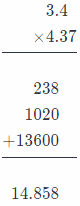So, the product is 14.858

Question 8.
3.762 × 0.66 = _______________
Here, in the first factor there are three decimal places, but in the second there are two, so, the product will has five decimal places.So, the product is 2.48292.

Question 9.
Chan Hee bought 3.4 pounds of coffee that cost $6.95 per pound. How much did he spend on coffee?$ ___________________
In order to calculate how much money Chan Hee spent on cotte, we need to multiply 3.4 by 6.95. First factor has one decimal place, but the second factor has two decimal places.
So, the product will has three decimal places.So, chan Hee spent 23.630 $on coffee. Question 10. Adita earns$9.40 per hour working at an animal shelter.
How much money will she earn for 18.5 hours of work? $_______________ Answer: In order to calculate how much money Adita will earn for 18.5 hours, we need to multiply 9.40 by 18.5. In the first factor there are two decimal places but in the second there is one decimal place. So, the product will has three decimal places: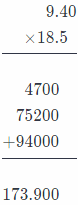So, Adita will earn 173.900$ for 18.5$hours of work. Catherin tracked her gas purchases for one month.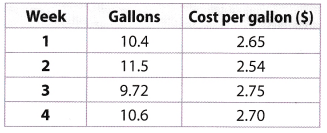Question 11. How much did Catherin spend on gas in week 2?$ ___________________________
In order to calculate how much Catherine spent on gas in week 2, we have to multiply 11.5 by 2.54 We can notice that result will has three decimal places: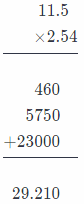Catherine spent 29.210 on gas in week 2$Question 12. How much more did she spend in week 4 than in week 1?$ ____________________________
First, we have to calculate how much Catherine spent on gas in week 1. we have to multiply 10.4 by 2.65. Here, the first factor has one decimal place hut the second one has two. the product will has three decimal places.Conclusion is that Catherine spent $27.560 on gas in week 1. Now. we will calculate how much Catherine spent on gas in week 4. We actually need to multiply 10.6 by 2.70. We can notice that time result will hase three decimal places: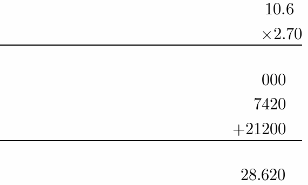We can see that Catherine spent$ 28.620 on gas in week 4.
Finally, we will subtract 27.560 from 28.620 in order to find how much more
Catherine spent on gas in week 4 than in week 1.
28.620 – 27.560 = 1.06
Catherine spent $1.06 more on gas in week 4 than in week 1. Essential Question Check-In Question 13. How can you check the answer to a decimal multiplication problem? Answer: We can check our answer to a decimal multiplication problem using the grid or draw an area model. Make a reasonable estimate for each situation. Question 14. A gallon of water weighs 8.354 pounds. Simon uses 11.81 gallons of water while taking a shower. About how many pounds of water did Simon use? Answer: In order to find how many pounds of water Simon used, we need to multiply 8.354 by 11.81. First factor has three decimals but the second one has two decimals, so, the result will has five decimalsSimon used 98.66074 pounds of water. Question 15. A snail moves at a speed of 2.394 inches per minute. If the snail keeps moving at this rate, about how many inches will it travel in 7.489 minutes? Answer: In order to calculate how many inches snail wilL travel in 7.489 minutes, we need to multiply 2.394 by 7.489. Both factors have three decimal places, so, the product will has six decimals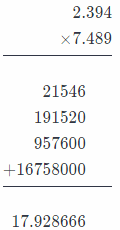Snail will travel 17.928666 inches if he keeps moving at this rate. Question 16. Tricia’s garden is 9.87 meters long and 1.09 meters wide. What is the area of her garden? Answer: In order to calculate the area of Tricia’s garden, we need to multiply 9.87 by 1.09. Both factors have two decimals, so, the product will has four decimal places.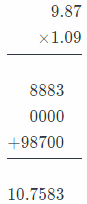So, the area of Tricia’s garden is 10.7583 square meters. Kaylynn and Amanda both work at the same store. The table shows how much each person earns, and the number of hours each person works in a week.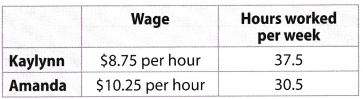Question 17. Estimate how much Kaylynn earns in a week. Answer: We will multiply 9, because 9 is closest to 8.75 by 37. So, we have the following: 9 × 37 = 333 So, kaylyum earns about 333$ per week

Question 18.
Estimate how much Amanda earns in a week.
We will multiply 10, because 10 is closest to 10.25 by 31. So, we have the following:
10 × 31 = 310
So, Amanda earns about 310$per week Question 19. Calculate the exact difference between Kaylynn and Amandas weekly salaries. Answer: We will multiply 8.75 by 37.5 in order to calculate Kaylyun’s weekly salanes. First factor has two decimals but the second one has decimal place, so, the product will has three decimals.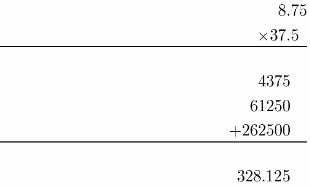So, Amanda earns$ 328.125 per week.
Now. we will calculate how much Amanda earns per week. So. we will multiply 10.25 by 30.5. Here, the first factor has two decimals but the second one has one decimal. so, the product will has three decimal places.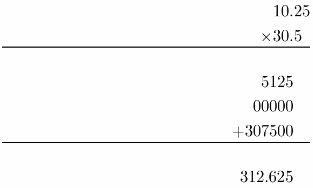So, Amanda earns $812.625 per week. Now, we will calculate the exact difference between Kaylyun and Amanda’s weekly salanes subtracting 312.625 from 328.125 and get: 328.125 – 312.625 = 15.5 So the exact difference between their salaries is$ 15.5.

Question 20.
Victoria’s printer can print 8.804 pages in one minute. If Victoria prints pages for 0.903 minutes, about how many pages will she have?
In order to calculate how many pages Victoria will have, we need to multiply 8.804 by 0.903. Both factors have three decimals, so, their product will have six decimals.So, Victoria will have 7.950012 pages.

A taxi charges a flat fee of $4.00 plus$2.25 per mile.

Question 21.
How much will it cost to travel 8.7 miles? ___________________
In order to calculate how much it will cost to travel 8.7 miles, we need to multiply 8.7 by 2.25 and on that product add 4 flat fee. First factor has one decimal but these can done has two decimals.
So, the product will have three decimals.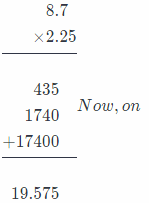19.575 we will add 4.00 and get:
19.574 + 4.00 = 23.575$So. it will cost$ 23.575 to travel 8.7 miles.

Question 22.
Multistep How much will the taxi driver earn if he takes one passenger 4.8 miles and another passenger 7.3 miles? Explain your process.
If taxi driver takes one passenger 4.8 miles, we will calculate how much he will earn in this case. We will first multiply 4.8 by 2.25 and on that product will add 4.00 flat fee.
The first factor has one decimal but the second has two decimals, so, the product will have three decimals.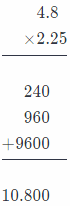Now, on 10.800 we will add 4.00 and get
10.800 + 4.00 = 14.800

So, it will cost 14.800 if taxi driver takes this passenger 4.8 miles. Now, we will calculate how much he will earn if he takes another passenger 73 miles. We will first multiply 73 by 2.25 and on that product we will add 4.00 flat fee. The first factor has on decimal but the second has two decimals, so, the product will have three decimals.16.425 we will add 4.00 and get:
16.425 + 4.00 = 20.425$So, it will cost$ 20.425 if taxi driver takes this passenger 7.3 mites.
If he takes both passengers, he will earn:
14.800 + 20.425 = 35.225
So, the taxi driver will earn 35.225$. Kay goes for several bike rides one week. The table shows her speed and the number of hours spent per ride.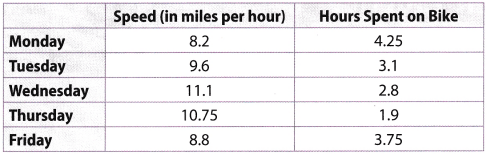Question 23. How many miles did Kay bike on Thursday? Answer: In order to calculate how many mites Kay biked on Thursday, we need to multiply 10.75 by 1.9. First factor has two decimals but the second has one, so, the product will have three decimals.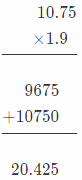Conclusion is that kay biked 20.425 miles on Thursday. Question 24. On which day did Kay bike a whole number of miles? Answer: We can notice that on Friday Kay biked a whole number of mites. Really, to calculate it, we need to multiply 8.8 by 3.75. The product will have three decimal places, so, we have the following:We can see that kay biked 33 miles on Friday. Question 25. What is the difference in miles between Kay’s longest bike ride and her shortest bike ride? Answer: Kay’s Longest bike ride was on Monday. Really, we need to multiply 8.2 by 4.25 in order to calculate length of this bike ride. We can notice that the product will have three decimals.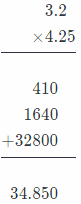So, Kay biked 34.850 miles on Monday. Her shortest bike ride was on Thursday, we already calculated it in the task 23. According to it, Kay biked 20.425 miles on Thursday. Now we will calculate the difference in miles between Kay’s longest and her shortest bike ride subtracting miles on Monday and miles on Thursday and get the following: 34.850 – 20.425 = 14.425 So, required difference was 14.425 mites. Question 26. Check for Reasonableness Kay estimates that Wednesday’s ride was about 3 miles longer than Tuesday’s ride. Is her estimate reasonable? Explain. Answer: Yes, her estimate is reasonable. We will estimate first Kay’s bike ride on Tuesday. In order to estimate it, we will multiply 10 by 3 and get: 10 × 3 = 30 Now, we will estimate Kay’s bike side on Wednesday. In order to estimate it, we will multiply 11 by 3 and get: 11 × 3 = 33. So, according to it, Kay’s reasonable was correct H.O.T. Higher Order Thinking Question 27. Explain the Error To estimate the product 3.48 × 7.33, Marisa multiplied 4 × 8 to get 32. Explain how she can make a closer estimate. Answer: She can make a closer estimate multiplying 3 by 7 because 3 is the closest whole number to 3.48 and 7 is the closest whole number to 7.33 Multiply 3 by 7 Question 28. Represent Real-World Problems A jeweler buys gold jewelry and resells the gold to a refinery. The jeweler buys gold for$1,235.55 per ounce, and then resells it for $1,376.44 per ounce. How much profit does the jeweler make from buying and reselling 73.5 ounces of gold? Answer: We will first calculate how much jeweler pays for 73.5 ounces of gold multiplying 1.235.55 by 73.5. The first factor has two decimals but the second one has one decimal, so, the product will have three decimals.So, the jeweler will pay$90,812.925.

Now, we will calculate how much he will get if he resells 73.5 ounces multiplying 1,376.44 by 73.5. The first factor has two decimals but the second one has one decimal, so, the product will have three decimals.So, he will get $101, 168.340 if he resells gold. Now, we wilt calculate how much profit the jeweler will make from bying and reselling subtracting 101, 168.340 and 90, 812.925 and get: 101, 168.340 – 90,812.925 = 10, 355.415 So, his profit will be$10, 355.415.

Question 29.
Problem Solving To find the weight of the gold in a 22 karat gold object, multiply the object’s weight by 0.916. To find the weight of gold in a 14 karat gold object, multiply the object’s weight by 0.585. Which contains more gold, a 22 karat gold object or a 14 karat gold object that each weigh 73.5 ounces? How much more gold does it contain?
First we will calculate contain of gold of 22 karat object multiplying the object’s weight, which is 73.5 ounces, by 0.916. The product will have four decimals:So, a 22 karat gold object contains 67.326 ounces of gold.
Now we will calculate contain of gold of 14 karat gold object multiplying the object’s weight, which is 73.5 ounces, by 0.585. The product will have four decimals: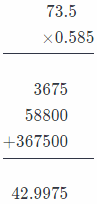So, a 14karat gold object contains 42.9975 ounces of gold.
We can see that more gold contains a 22 karat gold object.

Now we will calculate how much more gold it contains subtracting 42.9975 from 67.3260 and get:
67.3260 – 42.9975 = 24.3285
So, a 22 karat gold object contains 24.3285 ounces of gold more than a 14 karat gold object with equal weights.
a 22 karat object; 24.3285

Scroll to Top